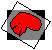# magnify

## Purpose:

This program will magnify images independently along the three major axes using Fourier interpolation. Magnification factors are restricted to values that can be expressed as the ratio of two integers, and the integer in the denominator must be a factor of the corresponding image dimension.

## Usage:

magnify input output overwrite?(y/n) magnify-x magnify-y magnify-z [shrink-x shrink-y shrink-z]

where the following definitions apply:
input
the name of the input file
output
the name of the output file
overwrite?(y/n)
'y' grants permission to overwrite output
magnify-x
the x magnification factor (an integer greater than or equal to 1
magnify-y
the y magnification factor (an integer greater than or equal to 1
magnify-z
the z magnification factor (an integer greater than or equal to 1
shrink-x
The optional x minification factor. This must be a positive integer and must be smaller than the x magnification factor (i.e., the net effect of the two factors cannot result in net minification). This value must also be a factor of the x dimension of the file.
shrink-y
The optional y minification factor. This must be a positive integer and must be smaller than the y magnification factor (i.e., the net effect of the two factors cannot result in net minification). This value must also be a factor of the y dimension of the file.
shrink-z
The optional z minification factor. This must be a positive integer and must be smaller than the z magnification factor (i.e., the net effect of the two factors cannot result in net minification). This value must also be a factor of the z dimension of the file.

## Examples:

magnify mri bigmri y 2 2 1
• The file "mri" will be transformed into a file "bigmri" which has the same number of planes as the input file, but has twice the x and y dimensions with voxels that are half as large. If the input x and y dimensions were 256, the corresponding output dimensions will be 512.
magnify mri bigmri y 3 3 1 2 2 1
• The file "mri" will be transformed into a file "bigmri" which has the same number of planes as the input file, but has 1.5 times the x and y dimensions with voxels that are 2/3 as large. If the input x and y dimensions were 256, the corresponding output dimensions will be 384.

• The use of Fourier interpolation results in near optimal resampling of the data at the larger size. Images can be expanded to several times their input size with acceptable looking results. Note that the interpolation assumes that the data wraps around in all dimensions. This is especially likely to cause problems along the z-axis where the newly generated slices at the bottom of the brain will start to take on characteristics of the top of the brain. You can use the program resize to clip off the extra planes created at the bottom if this is a problem.

## Error messages: (alphabetical by case)

Magnifications must be larger than corresponding shrinkages
• for example, shrink-x must be smaller than magnify-x
magnify_x must be a positive integer
• provide an integer greater than or equal to 1
magnify_y must be a positive integer
• provide an integer greater than or equal to 1
magnify_z must be a positive integer
• provide an integer greater than or equal to 1
shrink_x must be a positive integer
• provide an integer greater than or equal to 1 and smaller than magnify-x
shrink_y must be a positive integer
• provide an integer greater than or equal to 1 and smaller than magnify-y
shrink_z must be a positive integer
• provide an integer greater than or equal to 1 and smaller than magnify-zModified: December 18, 2001